# Hertz and Lenard’s Observations of the Photoelectric Effect

It was in 1887 when Heinrich Hertz was conducting experiments to prove Maxwell’s electromagnetic theory of light that he noticed a strange phenomenon. Hertz used a spark gap (two sharp electrodes placed at a small distance so that electric sparks can be generated) to detect the presence of electromagnetic waves. He placed it in a dark box to get a closer look and found that the spark length was reduced. When he used a glass box, the spark length increased, and when he replaced it with a quartz box, the spark length increased further. This was the first observation of the photoelectric effect.

A year later, Wilhelm Hallwachs confirmed these results and showed that UV light on a Zinc plate connected to a battery generated a current (because of electron emission). In 1898, J.J. Thompson found that the current amount varied with the radiation intensity and frequency.

In 1902, Lenard observed that the kinetic energy of electrons emitted increased with the frequency of radiation used. This could not be explained as Maxwell’s electromagnetic theory (which Hertz proved correct) predicted that the kinetic energy should depend only on light intensity (not frequency).

The resolution would only come a few years later by Einstein when he would provide an explanation of the photoelectric effect.

## Experimental Set Up

J.J. Thompson’s set-up (later improved by Lenard) to study this effect is of great importance. It consists of two zinc plate electrodes placed on the opposite ends of an evacuated (a vacuum is maintained) glass tube. A small quartz window illuminates one of the electrodes that is made the cathode. Quartz is used because ordinary glass blocks Ultra-Violet light. A battery and a potentiometer exert a variable voltage across the two electrodes. The current in the circuit can be recorded using an ammeter as the potential and light intensity change. The set-up is shown below: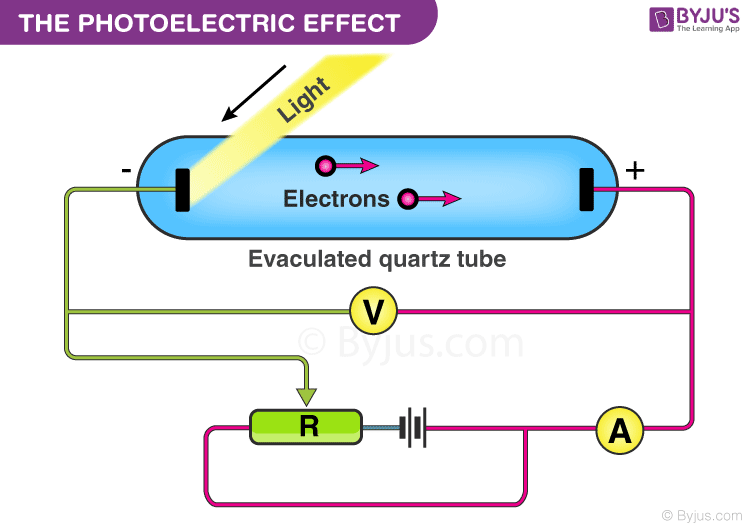### Observations

1. The photoelectric current (same as the rate of emission of electrons) is directly proportional to the intensity of light falling on the electrode. Note from the figure below that with increasing intensity, the current increases. Also, observe that as the voltage has decreased, the current also decreases. But to obtain zero current, the voltage has to be reversed to a certain V­0 known as the stopping potential. The voltage must be reversed so that the electrons cannot reach the anode. This is the maximum kinetic energy an emitted electron can achieve,

Maximum Kinetic energy,

$$\begin{array}{l}KE = eV_0\end{array}$$

(e is the charge of the electron)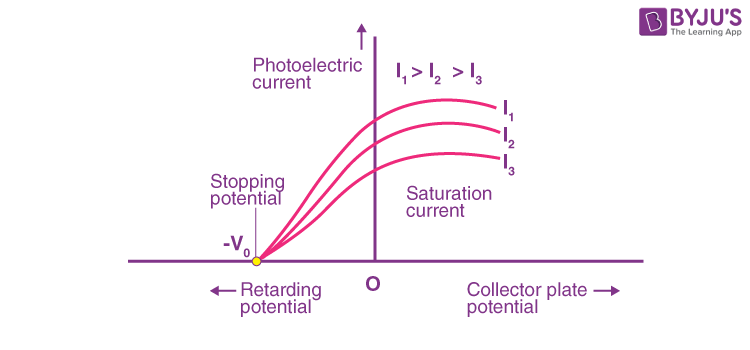Note that the stopping potential is independent of the intensity of light.

1. TThe maximum kinetic energy increases with an increase in the frequency of light. With a higher frequency of light (ν), the stopping potential becomes more negative, which implies that the kinetic energy of electrons also increases.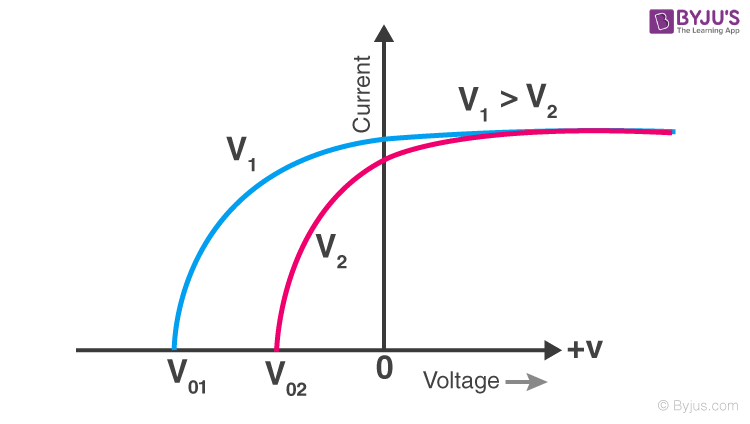1. All frequencies of light, however, cannot cause a photoelectric current to develop. Only light above a certain frequency (ν0) can produce a photoelectric current. This frequency is known as the threshold frequency. This varies with the electrode material. Also, the maximum kinetic energy of the electrons increases linearly with increasing light frequency. If we extend the graph below the x-axis, the intercept on the Kinetic energy axis represents the minimum energy required for the emission of the electron; this is known as the material’s work function.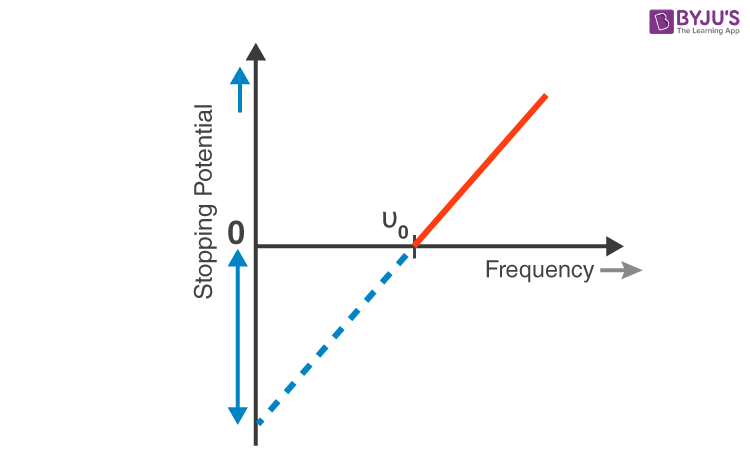2. Lastly, the electron emission occurs instantly without any time lag.

## Watch the video to learn about Hertz and Lenards Observation of the Photoelectric Effect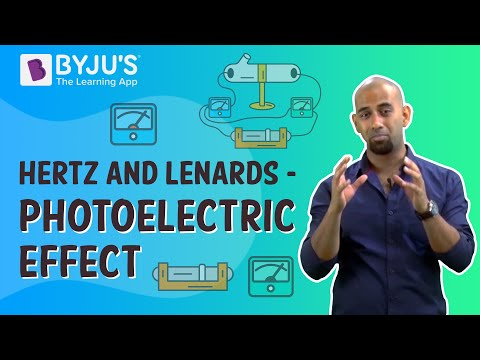## Frequently Asked Questions – FAQs

Q1

### What did Hertz observe about the photoelectric effect?

In 1887, Heinrich Hertz made the observation that when light strikes a metal surface, certain electrons close to the surface can absorb enough energy from the incoming radiation to overcome the attraction of the positive ions in the surface material.
Q2

### What does the term “threshold frequency” mean?

“Threshold frequency” is defined as the minimum frequency at which photoelectric emission is not possible.
Q3

### What factors affect threshold frequency?

The threshold frequency depends on the metal or material of the cathode.
Q4

### The photoelectric effect was it discovered by Einstein or Hertz?

German physicist Heinrich Rudolf Hertz made the discovery of the photoelectric effect in 1887.
Q5

### The two main observations are:

(i) The maximum kinetic energy of emitted photoelectrons is independent of light intensity.
(ii) For each photoelectron, there must be a threshold frequency of incident light below which no emission takes place.

To know how Albert Einstein explained these phenomena in his Nobel Prize-winning work, check out our article by visiting our site BYJU’S.

Test Your Knowledge On Hertz Lenard Observations!